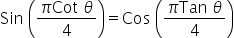Maths-
General
Easy

###Hint:

In this question, we have to find the value of theta if theta is in first quadrant in the equation. For this we will make trigonometric identity of both sides equal and then we will equate the sides to get the value of theta.

## Book A Free Demo+91